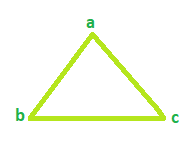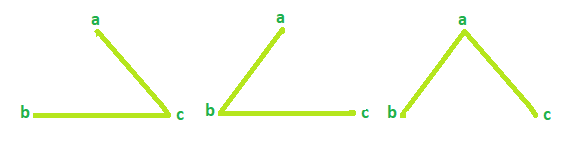# Total number of Spanning trees in a Cycle Graph

Given the number of vertices in a Cycle graph. The task is to find the Total number of Spanning trees possible.
Note: A cycle/circular graph is a graph that contains only one cycle. A spanning tree is a shortest/minimum path in a graph that covers all the vertices of a graph.

Examples:

```Input: Vertices = 3
Output: Total Spanning tree = 3

Input: Vertices = 4
Output: Total Spanning tree = 4
```

## Recommended: Please try your approach on {IDE} first, before moving on to the solution.

Example 1:
For Cycle Graph with vertices = 3Spanning Tree possible is 3Example 2:
For Cycle Graph with vertices = 4Spanning Tree possible is 4So, the number of spanning treess will always be equal to the number of vertices in a cycle graph.

Below is the required implementation:

## C++

 `// C++ program to find number of ` `// spanning trees ` `#include ` `using` `namespace` `std; ` ` `  `// function that calculates the ` `// total Spanning tree ` `int` `Spanning(``int` `vertices) ` `{ ` `    ``result = 0; ` ` `  `    ``result = vertices; ` `    ``return` `result; ` `} ` ` `  `// Driver code ` `int` `main() ` `{ ` `    ``int` `vertices = 4; ` ` `  `    ``cout << ``"Spanning tree = "` `<< Spanning(vertices); ` `    ``return` `0; ` `} `

## Java

 `// Java  program to find number of  ` `// spanning trees  ` ` `  `import` `java.io.*; ` ` `  `class` `GFG { ` ` `  `// function that calculates the  ` `// total Spanning tree  ` `static` `int` `Spanning(``int` `vertices)  ` `{  ` `    ``int` `result = ``0``;  ` ` `  `    ``result = vertices;  ` `    ``return` `result;  ` `}  ` ` `  `// Driver code  ` `    ``public` `static` `void` `main (String[] args) { ` `    ``int` `vertices = ``4``;  ` ` `  `    ``System.out.println(``"Spanning tree = "` `+ Spanning(vertices)); ` `    ``} ` `} ` `// This code is contributed   ` `// by chandan_jnu.. `

## Python3

 `# Python program to find number of  ` `# spanning trees  ` ` `  `# function that calculates the  ` `# total Spanning tree  ` `def` `Spanning( vertices): ` `        ``result ``=` `0` ` `  `    ``result ``=` `vertices  ` `    ``return` `result  ` ` `  `# Driver code  ` `vertices ``=` `4` `print``(``"Spanning tree = "``,  ` `       ``Spanning(vertices)) ` ` `  `# This code is contributed ` `# by Sanjit_Prasad `

## C#

 `// C# program to find number  ` `// of spanning trees ` `using` `System; ` ` `  `// function that calculates  ` `// the total Spanning tree ` `class` `GFG ` `{ ` `public` `int` `Spanning(``int` `vertices) ` `{ ` `    ``int` `result = 0; ` ` `  `    ``result = vertices; ` `    ``return` `result; ` `} ` ` `  `// Driver code ` `public` `static` `void` `Main() ` `{ ` `    ``GFG g = ``new` `GFG(); ` `    ``int` `vertices = 4; ` ` `  `    ``Console.WriteLine(``"Spanning tree = {0}"``,    ` `                      ``g.Spanning(vertices)); ` `} ` `} ` ` `  `// This code is contributed ` `// by Soumik `

## PHP

 ` `

Output:

```Spanning tree = 4
```

Don’t stop now and take your learning to the next level. Learn all the important concepts of Data Structures and Algorithms with the help of the most trusted course: DSA Self Paced. Become industry ready at a student-friendly price.

My Personal Notes arrow_drop_upCheck out this Author's contributed articles.

If you like GeeksforGeeks and would like to contribute, you can also write an article using contribute.geeksforgeeks.org or mail your article to contribute@geeksforgeeks.org. See your article appearing on the GeeksforGeeks main page and help other Geeks.

Please Improve this article if you find anything incorrect by clicking on the "Improve Article" button below.

Article Tags :
Practice Tags :

Be the First to upvote.

Please write to us at contribute@geeksforgeeks.org to report any issue with the above content.